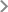﻿Programs-Central South University
CSU QR CodeSchoolsMathematics and StatisticsPrograms

## Mathematics and Statistics

Research areas for Master degree

Functional analysis

Algebra and combinatorics

Qualitative theory of differential equations

Numerical methods for differential equations

Financial Mathematics

Nonlinear partial differential equations

Graph theory

Research areas for PhD

Functional analysis

Algebra and combinatorics

Qualitative theory of differential equations

Numerical methods for differential equations

Financial Mathematics